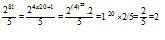# Sample Aptitude Questions of Mindtree

1. A cricketer played 80 innings and scored an average of 99 runs. His score in the last inning was zero run. To have an average of 100 at the end, his score in the last innings should have been
1. 60 runs
2. 80 runs
3. 10 runs
4. 1 run

Let x be the score in the last inning to make the average of 100
Therefore, when average is 99, total score of 80 innings = 80 × 99 = 7920
And when the average is 100, total score of 80 innings = 80 × 100 = 8000
Therefore, x = 8000 – 7920 = 80
2. A man spends an average of Rs. 1,694.70 per month for the first 7 months and Rs.1,810.50 per month for the next 5 months. His monthly salary if he saves Rs. 3,084.60 during the whole year is
1. Rs. 1000
2. Rs. 2000
3. Rs. 2400
4. Rs. 3000

Monthly Salary = [(1694.70*7)+(1810.5*5)+3084.60] / 12 = 24000/12 = 2000/-
1. A and B undertake to do a piece of work for Rs. 2,200. A alone can do it in 8 days, while B can do it in 6 days. With the help of C, they complete it in 3 days. Find C's share.
1. Rs. 150
2. Rs. 275
3. Rs. 245
4. Rs. 175

Let 1 be the total work
Therefore, C’s one day work = [1/3]-[1/8 + 1/6]= 1/24
Ratio of share of A, B and C
i.e. A:B:C = 1/8 : 1/6 :1/24 => 3 : 4 : 1
So, C’s share = 1/8*2200=275
2. By selling an article at 80% of its marked price, a trader makes a loss of 10%. What will be the profit percentage if he sells it at 95% of its marked price?
1. 5.9
2. 12.5
3. 6.9
4. 5

Let us assume the MP to be 900/-
Therefore, SP = 80% of MP = 0.80 × 900 = 720/-
As loss is 10%, so CP = 720/90 * 100=800
Now, if SP = 95% of MP = 0.95 × 900 = 855/-
Profit% = [{855-800}/800 ]*100 =6.87
3. By selling an umbrella for Rs. 30, a shopkeeper gains 20%. During a clearance sale, the shopkeeper allows a discount of 10% of the marked price. His gain during the sale season is
1. 8
2. 9
3. 7
4. 7.5

Given that SP of umbrella = 30/- and profit% = 20.
Therefore, CP of umbrella = 30/120 *100=25
SP of umbrella after 10% discount = 90/100 *30= 27
Thus, profit% = {(27-25) /25 }*100=8
4. The Arithmetic mean of 5, 10, 12, 18, 20 is:
1. 5
2. 65
3. 13
4. 12

Arithmetic mean= 5+10+12+18+20/5=65/5=13
5. From each of two given numbers, half the smaller number is subtracted. After such subtraction, the larger number is 4 times as large as the smaller number. What is the ratio of the numbers?
1. 4:1
2. 4:5
3. 5:2
4. 1:4

Let x and y be the two numbers, with x > y
Now are given that x -y/2=4[y - y/2]
Solving the above equation, we get x/y = 5:2
6. What is the remainder if 281 is divided by 5?
1. 4
2. 1
3. 2
4. 37. What is the largest number which divides 150, 180 and 144 leaving the same remainder in each case?
1. 12
2. 3
3. 6
4. 8

HCF (180-150,180-144,150-144)
HCF(30,36,6)=6
8. In an examination, 52% of the candidates failed in English and 43% failed in Mathematics. If 17% failed in both the subjects, then the percentage of candidates, who passed in both the subjects, was
1. 25
2. 22
3. 23
4. 21